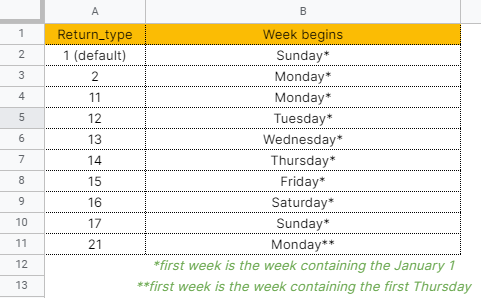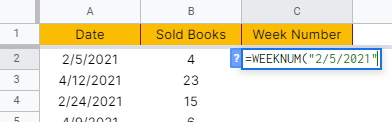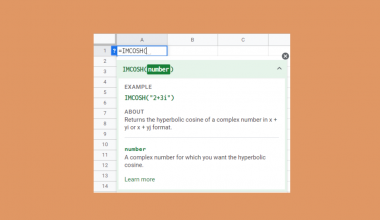# How to Use WEEKNUM Function in Google Sheets

The WEEKNUM function in Google Sheets is useful if you want to know the week number of a specific date.

Meaning, the WEEKNUM function returns a week number between 1-52, which corresponds to the week of the year.

The rules for using the WEEKNUM function in Google Sheets are as follows:

• If no value is passed to the second argument, return_type, it will take the default value of 1.
• The serial_number argument for the WEEKDAY function should be entered as either a reference to a cell containing a date or a date returned from another function or formula.
• The function will return a #VALUE! error if the given serial_number is non-numeric or cannot be recognized as a valid date.
• The function will return a #NUM! error if the value passed to return_type argument is not the value permitted by the function.

Let’s take an example.

To be able to create a good summary report, Prim would like to get the total number of books sold per week. See her table below:First, she needs to identify what week number each date falls. Afterward, she can proceed to create a pivot table to able to aggregate the numbers for each week.

Using the WEEKNUM function, Prim was able to yield the week number each date falls. See her updated table below:She went ahead and created the pivot table to get the aggregated sold books per week:Pretty smart, right?

Watch out for a more advanced tutorial and examples on how you can use the WEEKNUM function in the coming weeks. Be sure to subscribe to be notified.

Perfect! Let’s begin getting to know more about our WEEKNUM function in Google Sheets.

## The Anatomy of the WEEKNUM Function

So the syntax (the way we write) the WEEKNUM function is as follows:

```=WEEKNUM(serial_number,[return_type])
```

Let’s dissect this thing and understand what each of these terms means:

• = the equal sign is just how we start any function in Google Sheets. It is how Google Sheets understand that we are asking it to either do computation or use a function.
• WEEKNUM() this is our WEEKNUM function. It gets the week number for a given date.
• serial_number is the week in which we want to return the week number.
• return_type are the text values that you wish to combine or join together.

## A Real Example of Using WEEKNUM Function

Let’s take a look at the report that Prim’s created below to see how the WEEKNUM function is used in Google Sheets.Notice in the formula used for each row that there’s only one argument passed to the WEEKNUM function.

Why didn’t the function return an error?

It is because the WEEKNUM function will consider the default value of 1 to the return_type argument if no value is provided.

Refer to the table below for the values that you may use as the second argument:Again, in the example above, Prim didn’t pass any value to the second argument, which means it will take Sunday as day 1 of the week.She gets the result below:We mentioned that not providing a value to the second argument is the same as providing 1 as the second argument.See the same result below:Also, note that if the first argument is not a reference to a cell where the date value is located, you must enclose the direct value in the WEEKNUM function with the quotation marks.

Otherwise, you will get an incorrect result. See sample below:You’ll get the incorrect result below:The date or the first argument should be enclosed between quotation marks for the function to interpret is as the date value:See the correct result below:You may make a copy of the spreadsheet using the link I have attached below.

## How to Use WEEKNUM Function in Google Sheets

1. Click on any cell to make it the active cell. For this guide, I will be selecting C2, where I want to show the resulting week number.1. Next, type the equal sign ‘=‘ to begin the function and then follow it with the name of the function, which is our ‘weeknum‘ (or ‘WEEKNUM‘, not case sensitive like our other functions).1. Type open parenthesis ‘(‘ or simply hit Tab key to let you use that function.1. Now the exciting part! Let’s give our function its first argument, the serial_number. Type in a quotation mark () and followed it with the date value, which is ‘2/5/2021’. Don’t forget to close the date value with another quotation mark ().1. To let the Google sheet know that we’re done typing our first argument, we should now type in the delimiter or the character that separates each argument on a function. In this case, type comma,’.1. Type in our second argument, which is the return_type. Type in ‘1’.1. Finally, hit your Enter or Tab key. Cell C2 will now show you the week number or the return value of the WEEKNUM function.1. Notice that in this example, we provided the exact value, constant, as the arguments of our WEEKNUM function. Alternatively, these constants can be variable or simply cell addresses of your data.

1. Edit your function by changing the first argument into the cell address where your serial_number is located. In this case, type in cell A2.1. Copy the formula down to the remaining rows.That’s pretty much it. You can now use the WEEKNUM function in Google Sheets together with the other numerous Google Sheets formulas to create even more powerful formulas that can make your life much easier.Our goal this year is to create lots of rich, bite-sized tutorials for Google Sheets users like you. If you liked this one, you'll love what we are working on! Readers receive ✨ early access ✨ to new content.

##### You May Also Like## How to Use IMCOSH Function in Google Sheets

The IMCOSH function in Google Sheets is useful when you need to display the hyperbolic cosine of a…## How to Fix ‘Formula Parse’ Error in Google Sheets

A formula parsing error message appears when the formula inputted expects a specific data type, but it received…## How to Use DSTDEVP Function in Google Sheets

The DSTDEVP function in Google Sheets is useful if you need to calculate the standard deviation of a…## How to Use the DAY Function in Google Sheets

The DAY function in Google Sheets is useful to return the day of the month that a specific…